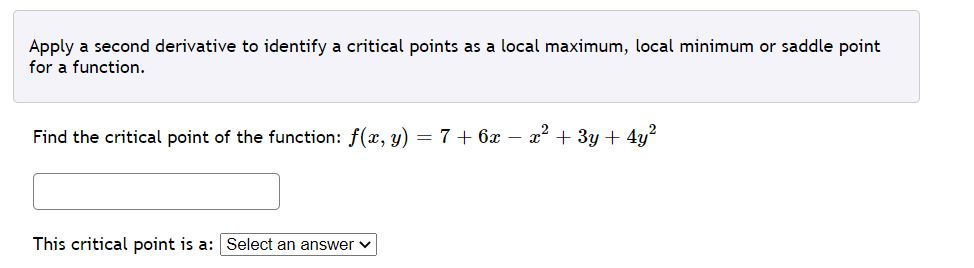# Question Solved1 Answer Apply a second derivative to identify a critical points as a local maximum, local minimum or saddle point for a function. Find the critical point of the function: f(x, y) = 7 + 6x - 2? + 3y + 4y? This critical point is a: Select an answer Apply a second derivative to identify a critical points as a local maximum, local minimum or saddle point for a function. Find the critical point of the function: f(a, y) = 7 + 6x — x” + 3y + 4y? This critical point is a:# Apply a second derivative to identify a critical points as a local maximum, local minimum or...Apply a second derivative to identify a critical points as a local maximum, local minimum or saddle point for a function. Find the critical point of the function: f(x, y) = 7 + 6x - 2? + 3y + 4y? This critical point is a: Select an answer
Transcribed Image Text: Apply a second derivative to identify a critical points as a local maximum, local minimum or saddle point for a function. Find the critical point of the function: f(a, y) = 7 + 6x — x” + 3y + 4y? This critical point is a:
More
Transcribed Image Text: Apply a second derivative to identify a critical points as a local maximum, local minimum or saddle point for a function. Find the critical point of the function: f(a, y) = 7 + 6x — x” + 3y + 4y? This critical point is a: